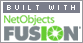Coming to Statistica
in 2022/2023

Zoom tutorials
using Explaineverything app
We a record sessions for you to listen to later

CALL STATISTICA NOW

.

E-mail     anne@statistica.com.au

# Place         Willetton, Western Australia# Mathematics & Statistic Tutor Perth - SPSS Help

Solutions to Tutorial Two          Descriptive statistics for Independent/Dependent data

Solutions to Tutorial Three     Boxplots by hand calculations, transformations, Stem and leaf plots

Solutions to Tutorial Four       Linear Regression

Solutions to Tutorial Five        Normal Distribution with individual values Z-Tables

Solutions to Tutorial Six          Sampling Distributions Normality Tests

Solutions to Tutorial Seven     Central Limit Theorem, Normal Distribution average values

Solutions to Tutorial Eight      Confidence Intervals for the population mean

Solutions to Tutorial Nine       Tests of significance  One sample with SPSS

Help with t-tables                     Critical values, probabilities involving t

Help with p-values                    Calculating p-value using SPSS and t-tables

Solutions to Tutorial Ten         Tests of significance Independent Dependent with SPSS

T-tests using Formulae            Tests of significances using formulae by hand[Home] [Parents] [Contact us] [Services] [Mathematics] [Statistics] [School]